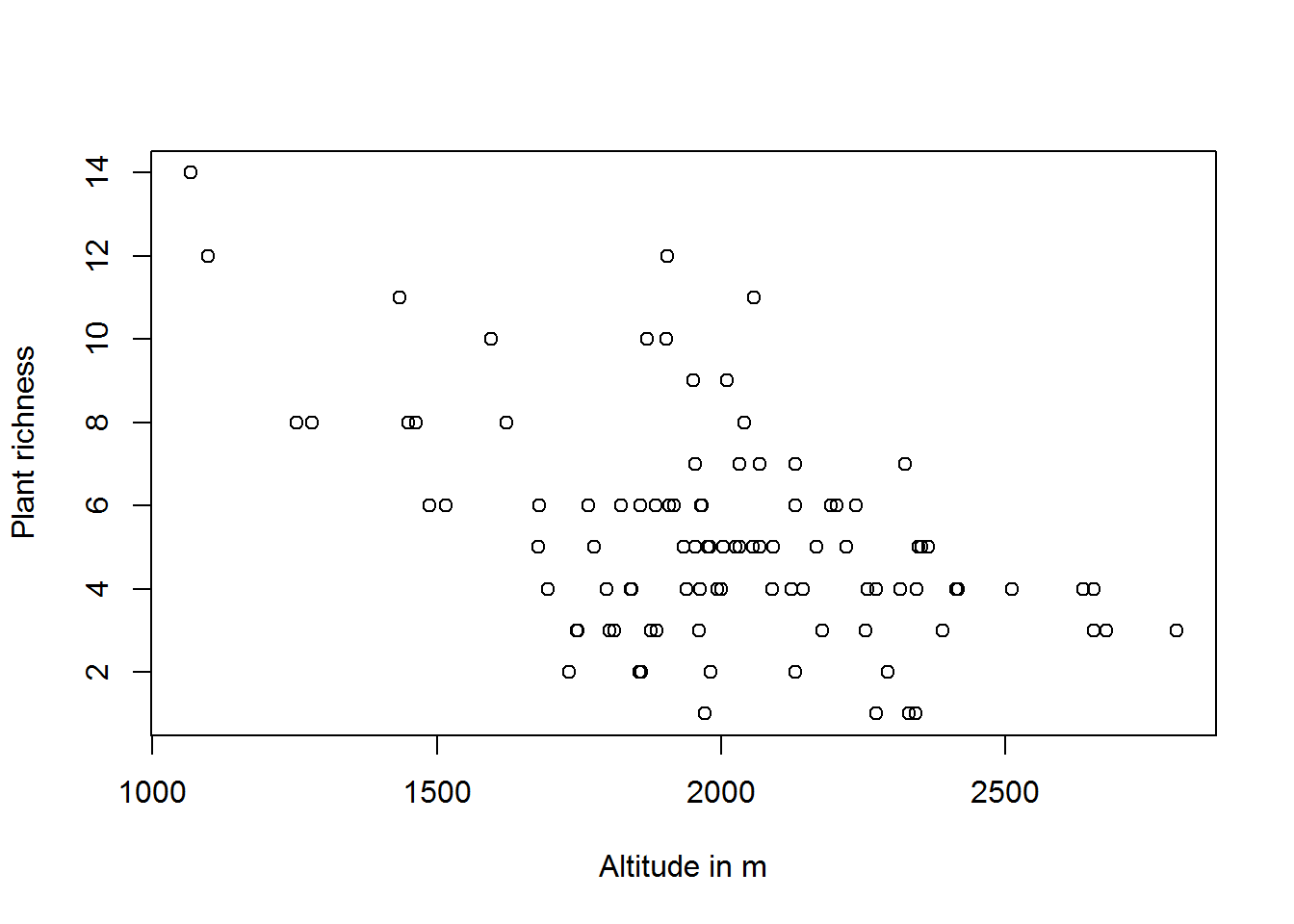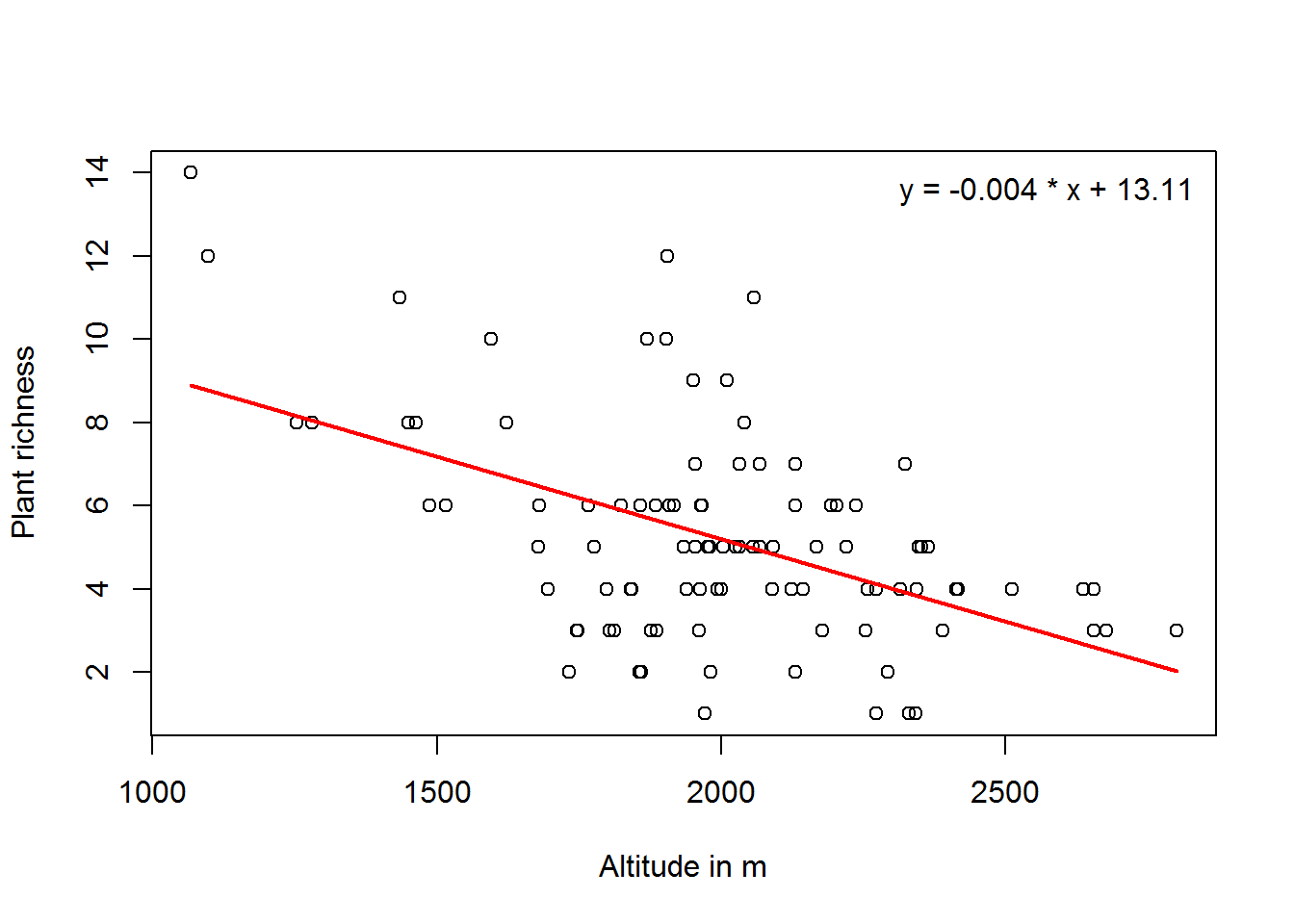BIS-Fogo

Site Tools

en:learning:schools:s01:code-examples:ba-ce-04-01

C04-1 Linear model

The following example uses data from a field survey of areas in the Fogo natural park in 2007 by K. Mauer. For more information, please refer to this report.

Scatterplot

To visualize the dependence between two variables, a scatterplot should be the first choice.

The following example illustrates the dependence between plant richness and elevation using the plot() function.

plot(data_2007$ALT_GPS_M, richness,xlab="Altitude in m",ylab="Plant richness")Although not a hard one, some kind of linear relationship can be observed with decreasing plant richness with altitude. Linear model Computation To model the relationship between two linearily related variables, a simple linear regression can be used. The following example illustrates the computation of a linear model between plant richness and elevation. linear_model <- lm(richness ~ data_2007$ALT_GPS_M)
print(linear_model)
##
## Call:
## lm(formula = richness ~ data_2007$ALT_GPS_M) ## ## Coefficients: ## (Intercept) data_2007$ALT_GPS_M
##            13.10981             -0.00396

The resulting model has an intercept of about 13.1 and a slope of about -0.01, i.e. decreasing plant richness with elevation. To visualize that, we can add the regression line to the scatter plot using the regline() function ( in addition we add the regression equation as a legend).

plot(data_2007$ALT_GPS_M, richness,xlab="Altitude in m",ylab="Plant richness") regLine(linear_model) legend("topright",legend=paste0("y = ", round(linear_model$coefficients,3),
" * x + ",
round(linear_model$coefficients,3)),bty="n")Goodness of fit To estimate the goodness of fit of a linear model, one can have a look at the coefficient of determination along with the p-value. summary(linear_model) ## ## Call: ## lm(formula = richness ~ data_2007$ALT_GPS_M)
##
## Residuals:
##    Min     1Q Median     3Q    Max
##  -4.30  -1.45  -0.04   1.23   6.44
##
## Coefficients:
##                      Estimate Std. Error t value Pr(>|t|)
## (Intercept)         13.109812   1.448783    9.05  1.5e-14 ***
## data_2007\$ALT_GPS_M -0.003961   0.000716   -5.53  2.7e-07 ***
## ---
## Signif. codes:  0 '***' 0.001 '**' 0.01 '*' 0.05 '.' 0.1 ' ' 1
##
## Residual standard error: 2.3 on 97 degrees of freedom
## Multiple R-squared:  0.24,   Adjusted R-squared:  0.232
## F-statistic: 30.6 on 1 and 97 DF,  p-value: 2.72e-07

One can see from the summary, that the model is highly significant with a p-value of much less than 0.01. Looking at an r squared of about 0.23, the model explains about 23% of the variability of the data set, i.e. one can explain 23% of the observed plant richness by the elevation of the observational plots.